Bihar Board 12th Maths Objective Questions and Answers

## Bihar Board 12th Maths Objective Answers Chapter 9 Differential Equations

Question 1.
The order of the differential equation of all tangent lines to the parabola y = x2 is
(a) 1
(b) 2
(c) 3
(d) 4
(a) 1

Question 2.
The differential equation of all parabolas whose axis of symmetry is along the axis of the x-axis is of order
(a) 3
(b) 1
(c) 2
(d) none of these
(c) 2

Question 3.
The degree of the equation satisfying the relation $$\sqrt{1+x^{2}}+\sqrt{1+y^{2}}=\lambda(\sqrt{1+y^{2}}-y \sqrt{1+x^{2}})$$ is
(a) 1
(b) 2
(c) 3
(d) none of these
(a) 1

Question 4.
The degree of the differential equation $$\left(\frac{d^{2} y}{d x^{2}}\right)^{2 / 3}+4-\frac{3 d y}{d x}=0$$ is
(a) 2
(b) 1
(c) 3
(d) none of these
(a) 2

Question 5.
The differential equation whose solution is (x – h)2 + (y – k)2 = a2 is (a is a constant)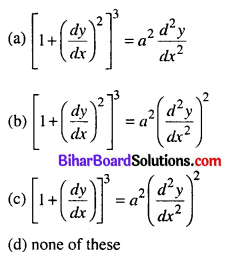(b) $$\left[1+\left(\frac{d y}{d x}\right)^{2}\right]^{3}=a^{2}\left(\frac{d^{2} y}{d x^{2}}\right)^{2}$$

Question 6.
The differential equation satisfied by y = $$\frac{A}{x}$$ + B is (A, B are parameters)
(a) x2 y1 = y
(b) xy1 + 2y2 = 0
(c) xy2 + 2y1 = 0
(d) none of these
(c) xy2 + 2y1 = 0

Question 7.
The differential equation whose solution represents the family xy = Aeax + Be-ax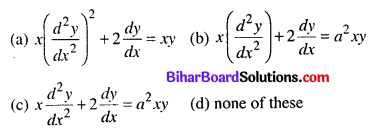(c) $$x \frac{d^{2} y}{d x^{2}}+2 \frac{d y}{d x}=a^{2} x y$$

Question 8.
The differential equation having solution is y = 17ex + ae-x is
(a) y” – x = 0
(b) y” – y = 0
(c) y’ – y = 0
(d) y’ – x = 0
(b) y” – y = 0

Question 9.
The solution of a differential equation is y = c1e4x + c2e3x, the differential equation is given by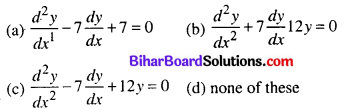(c) $$\frac{d^{2} y}{d x^{2}}-7 \frac{d y}{d x}+12 y=0$$

Question 10.
The differential equation satisfied by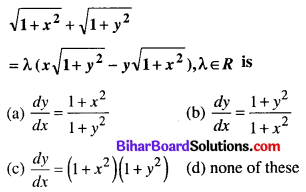(b) $$\frac{d y}{d x}=\frac{1+y^{2}}{1+x^{2}}$$

Question 11.
The differential equation of all ‘Simple Harmonic Motions’ of given period $$\frac{2 \pi}{n}$$ is(b) $$\frac{d^{2} x}{d t^{2}}+n^{2} x=0$$

Question 12.
The differential equation of all parabolas whose axes are parallel to y-axis is(a) $$\frac{d y}{d x}=-\frac{c^{2}}{x^{2}}$$

Question 13.
The differential equation of all non-horizontal lines in a plane is(b) $$\frac{d^{2} x}{d y^{2}}=0$$

Question 14.
The differential equation of all circles which pass through the origin and whose centre lies on y-axis is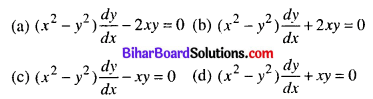(a) $$\left(x^{2}-y^{2}\right) \frac{d y}{d x}-2 x y=0$$

Question 15.
The differential equation of the family of circles touching the x-axis at origin is given by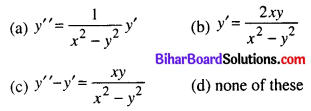(b) $$y^{\prime}=\frac{2 x y}{x^{2}-y^{2}}$$

Question 16.
The differential equation representing the family of ellipses with centre at origin and foci on x-axis is given as
(a) xy’ + y = 0
(b) x2y2(y”)2 + yy’= 0
(c) xyy” + x(y’)2 – yy’ = 0
(d) None of these
(b) x2y2(y”)2 + yy’= 0

Question 17.
The differential equation of all parabolas whose axes are along x-axis is
(a) $$y_{2}^{2}+y_{1}=0$$
(b) $$y_{1}^{2}+y_{2}=0$$
(c) $$y_{1}^{2}+y_{1} y_{2}=0$$
(d) $$y_{1}^{2}+y y_{2}=0$$
(d) $$y_{1}^{2}+y y_{2}=0$$

Question 18.
The equation of family of curves for which the length of the normal is equal to the radius vector is
(a) $$y^{2} \mp x^{2}=k^{2}$$
(b) $$y \pm x=k$$
(c) y2 = kx
(d) none of these
(a) $$y^{2} \mp x^{2}=k^{2}$$

Question 19.
The solution of the differential equation $$\frac{d y}{d x}=\frac{x^{2}+y^{2}+1}{2 x y}$$ satisfying (1) = 1, is
(a) a hyperbola
(b) a circle
(c) y2 = x(1 + x) – 10
(d) (x – 2)2 + (y – 3)2 = 5xy
(a) a hyperbola

Question 20.
Given the differential equation $$\frac{d y}{d x}=\frac{6 x^{2}}{2 y+\cos y}$$; y(1) = π
Mark out the correct statement.
(a) solution is y2 – sin y = -2x3 + C
(b) solution is y2 + sin y = 2x3 + C
(c) C = π2+ 2√2
(d) C = π2 + 2
(b) solution is y2 + sin y = 2x3 + C

Question 21.
For the differential equation $$x \frac{d y}{d x}+2 y=x y \frac{d y}{d x}$$,
(a) order is 1 and degree is 1
(b) solutio is ln(yx2) = C – y
(c) order is 1 and degree is 2
(d) solution is ln(xy2) = C + y
(a) order is 1 and degree is 1

Question 22.
The particular solution In($$\frac{d y}{d x}$$) = 3x + 4y, y(0) = 0 is
(a) e3x + 3e-4y = 4
(b) 4e3x – 3e-4y = 3
(c) 3e3x + 4e4y = 7
(d) 4e3x + 3e-4y = 7
(d) 4e3x + 3e-4y = 7

Question 23.
The solution of the differential equation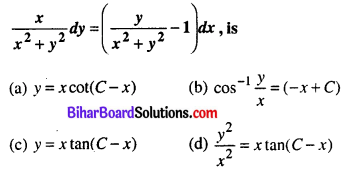(c) y = x tan(C – x)

Question 24.
The solution of the differential equation(d) None of these

Question 25.
The solution of the differential equation(c) $$y=x \tan \left(\frac{C-x^{2}-y^{2}}{2}\right)$$

Question 26.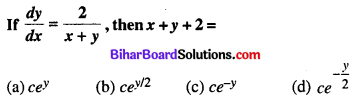(b) $$c e^{y / 2}$$

Question 27.
If ydx – xdy + ln x dx = 0, y(1) = -1, then
(a) y + 1 + ln x = 0
(b) y + 1 + 2 ln x = 0
(c) 2(y + 1) + lnx = 0
(d) y + 1 – y ln x = 0
(a) y + 1 + ln x = 0

Question 28.
The differential equation $$\frac{d y}{d x}=\sqrt{\frac{1-y^{2}}{y}}$$ determines a family of circle with
(a) variable radii and fixed centre (0, 1)
(b) variable radii and fixed centre (0, -1)
(c) fixed radius 1 and variable centre on x-axis
(d) fixed radius 1 and variable centre on y-axis
(c) fixed radius 1 and variable centre on x-axis

Question 29.
If y dx + y2 dy = x dy, x ∈ R, y > 0 and y(1) = 1, then y(-3) =
(a) 3
(b) 2
(c) 1
(d) 5
(a) 3

Question 30.
The solution of y dx + (x + x2y) dy = 0 is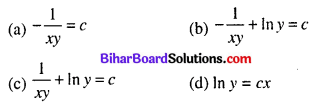(b) $$-\frac{1}{x y}+\ln y=c$$

Question 31.
If (x + y)2 $$\frac{d y}{d x}$$ = a2, y = 0 when x = 0, then y = a if $$\frac{x}{a}$$ =
(a) 1
(b) tan 1
(c) tan 1 + 1
(d) tan 1 – 1
(d) tan 1 – 1

Question 32.(a) ex – 1

Question 33.
If sinx $$\frac{d y}{d x}$$ + y cosx = x sinx, then (y – 1) sinx =
(a) c – x sinx
(b) c + xcosx
(c) c – x cos x
(d) c + x sin x
(c) c – x cos x

Question 34.
The solution of the differential equation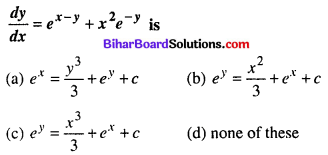(c) $$e^{y}=\frac{x^{3}}{3}+e^{x}+c$$

Question 35.
The solution of the differential equation xdy + ydx = xydx when y(1) = 1 is(b) $$\frac{e^{x}}{e x}$$

Question 36.
The solution of differential equation (ey + 1) cosx dx + ey sinx dy = 0 is
(a) (ey + 1) sinx = c
(b) ex sinx = c
(c) (ex + 1) cosx = c
(d) none of these
(a) (ey + 1) sinx = c

Question 37.
The solution of the differential equation $$\frac{d y}{d x}=\frac{x}{1+x^{2}}$$ is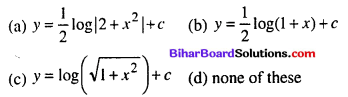(c) $$y=\log (\sqrt{1+x^{2}})+c$$

Question 38.(c) $$\frac{e^{6}+9}{2}$$

Question 39.(a) y = e sin2x

Question 40.
The general solution of the differential equation $$\frac{d y}{d x}=\frac{x^{2}}{y^{2}}$$ is
(a) x3 – y3 = c
(b) x3 + y3 = c
(c) x2 + y2 = c
(d) x2 – y2 = c
(a) x3 – y3 = c

Question 41.
The Solution of cos(x + y) dy = dx is(a) $$y=\tan \left(\frac{x+y}{2}\right)+C$$

Question 42.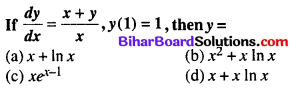(d) x + x ln x

Question 43.(c) √3e

Question 44.(d) $$\ln \frac{y}{x}=c x$$

Question 45.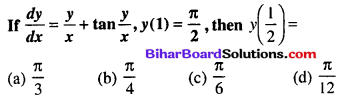(d) $$\frac{\pi}{12}$$

Question 46.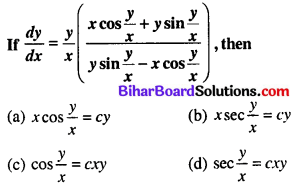(d) $$\sec \frac{y}{x}=c x y$$

Question 47.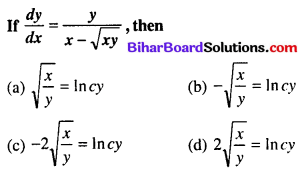(c) $$-2 \sqrt{\frac{x}{y}}=\ln c y$$

Question 48.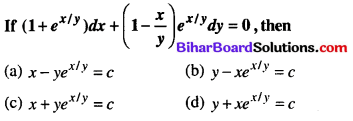(c) $$x+y e^{x / y}=c$$

Question 49.(a) $$x y=c e^{y / x}$$

Question 50.(c) Circle

Question 51.(c) $$\sqrt{x^{2}+y^{2}}+y=c x^{2}$$

Question 52.
The solution of the differential equation (x2 + y2) dx – 2xy dy = 0 is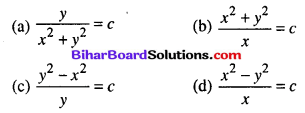(d) $$\frac{x^{2}-y^{2}}{x}=c$$

Question 53.
The solution of the differential equation x dy + (x + y) dx = 0 is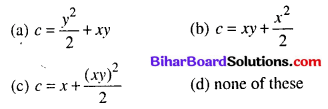(b) $$c=x y+\frac{x^{2}}{2}$$

Question 54.
The solution of differential equation $$\frac{d y}{d x}=\frac{x-y}{x+y}$$ is
(a) x2 – y2 + 2xy + c = 0
(b) x2 – y2 – xy + c = 0
(c) x2 – y2 + xy + c = 0
(d) x2 – y2 – 2xy + c = 0
(d) x2 – y2 – 2xy + c = 0

Question 55.(a) $$-\frac{1}{2}$$

Question 56.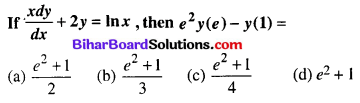(c) $$\frac{e^{2}+1}{4}$$

Question 57.(d) 6

Question 58.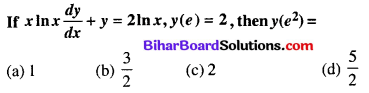(d) $$\frac{5}{2}$$

Question 59.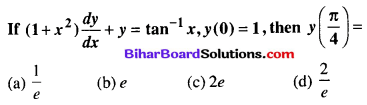(d) $$\frac{2}{e}$$

Question 60.(d) $$\frac{1}{4}$$

Question 61.(c) $$\frac{x}{y}-y^{2}=c$$

Question 62.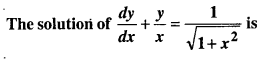(b) $$y=\frac{\sqrt{1+x^{2}}}{x}+\frac{c}{x}$$

Question 63.(c) $$y e^{-3 x}=-e^{-3 x} \frac{(2 \cos 2 x+3 \sin 2 x)}{13}+c$$

Question 64.(a) $$-\frac{1}{2}$$

Question 65.
The solution of the differential equation,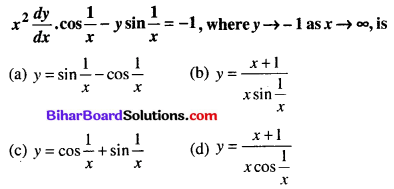(a) $$y=\sin \frac{1}{x}-\cos \frac{1}{x}$$

Question 66.
The degree of the differential equation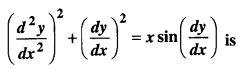(a) 1
(b) 2
(c) 3
(d) not defined
(d) not defined

Question 67.
The order and degree of the differential equation $$\frac{d^{2} y}{d x^{2}}+\left(\frac{d y}{d x}\right)^{\frac{1}{4}}+x^{\frac{1}{5}}=0$$ respectively are
(a) 2 and not defined
(b) 2 and 2
(c) 2 and 3
(d) 3 and 3
(a) 2 and not defined

Question 68.
The differential equation for y = A cos αx + B sin αx, where A and B are arbitrary constants is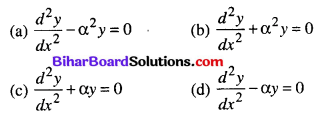(b) $$\frac{d^{2} y}{d x^{2}}+\alpha^{2} y=0$$

Question 69.
Integrating factor of the differential equation(c) $$\sqrt{1-x^{2}}$$

Question 70.
Integrating factor of the differential equation $$\frac{d y}{d x}$$ + y tanx – sec x = 0 is
(a) cos x
(b) sec x
(c) ecos x
(d) esec x
(b) sec x

Question 71.
The solution of the differential equation $$\frac{d y}{d x}=\frac{1+y^{2}}{1+x^{2}}$$ is
(a) y = tan-1 x
(b) y – x = k(1 + xy)
(c) x = tan-1 y
(d) tan(xy) = k
(b) y – x = k(1 + xy)

Question 72.
The solution of the differential equation cos x sin y dx + sin x cos y dy = 0 is
(a) $$\frac{\sin x}{\sin y}=c$$
(b) sin x sin y = c
(c) sin x + sin y = c
(d) cos x cos y = c
(b) sin x sin y = c

Question 73.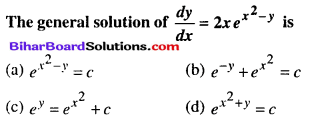(c) $$e^{y}=e^{x^{2}}+c$$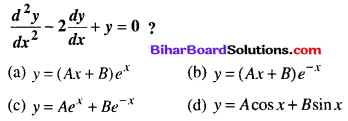(a) $$y\left(1+x^{2}\right)=c+\tan ^{-1} x$$